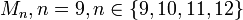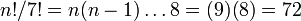# Mathieu group:M9

View a complete list of particular groups (this is a very huge list!)[SHOW MORE]

## Definition

This group, denoted$M_9$, and termed the Mathieu group of degree nine, is defined in the following equivalent ways:$\! M_9 := \langle (1,4,9,8)(2,5,3,6), (1,6,5,2)(3,7,9,8) \rangle$.

This is one of the Mathieu groups, but is not one of the five sporadic simple Mathieu groups. Rather, it is among the two Mathieu groups (the other being Mathieu group:M10) that are not simple. The Mathieu group$M_{21}$ is simple, but not a sporadic simple group -- it is isomorphic to projective special linear group:PSL(3,4).

## Arithmetic functions

Function Value Similar groups Explanation
order (number of elements, equivalently, cardinality or size of underlying set) 72 groups with same order As semidirect product of two-dimensional vector space over field:F3 by quaternion group:$3^2 \cdot 8 = 72$. See order of semidirect product is product of orders
As Mathieu group$M_n, n = 9, n \in \{ 9,10,11,12 \}$:$n!/7! = n(n-1) \dots 8 = (9)(8) = 72$
As$\! PSU(n,q)$,$\! n = 3, q = 2$: PLACEHOLDER FOR INFORMATION TO BE FILLED IN: [SHOW MORE]
exponent of a group 12 groups with same order and exponent of a group | groups with same exponent of a group Elements of order$1,2,3,4$.
nilpotency class not a nilpotent group.
derived length 3 groups with same order and derived length | groups with same derived length
Frattini length 1 groups with same order and Frattini length | groups with same Frattini length Frattini-free group
Fitting length 2 groups with same order and Fitting length | groups with same Fitting length
minimum size of generating set 2 groups with same order and minimum size of generating set | groups with same minimum size of generating set Two elements of order four from different$2$-Sylow subgroups.
subgroup rank of a group 2 groups with same order and subgroup rank of a group | groups with same subgroup rank of a group
max-length of a group 5 groups with same order and max-length of a group | groups with same max-length of a group

### Arithmetic functions of a counting nature

Function Value Explanation
number of subgroups 68 See subgroup structure of Mathieu group:M9
number of conjugacy classes 6
number of conjugacy classes of subgroups 14 See subgroup structure of Mathieu group:M9

## Group properties

Property Satisfied Explanation Comment
Abelian group No
Nilpotent group No
Supersolvable group No
Solvable group Yes
T-group No The$3$-Sylow subgroup is abelian normal, has subgroups that are not normal.
Monolithic group Yes Unique minimal normal subgroup is$3$-Sylow subgroup.
One-headed group No Three maximal normal subgroups of order$36$.
Rational group Yes
Rational-representation group No Its quotient, quaternion group, is not a rational-representation group.
Ambivalent group Yes
Any two elements generating the same cyclic subgroup are automorphic Yes Follows from being a rational group
Every element is automorphic to its inverse Yes Follows from being an ambivalent group
Frobenius group Yes Frobenius kernel is$3$-Sylow subgroup, complement is$2$-Sylow subgroup
Camina group Yes Derived subgroup is of order$18$, three non-identity cosets are conjugacy classes.

## Linear representation theory

Further information: linear representation theory of Mathieu group:M9

### Summary

Item Value
Degrees of irreducible representations over a splitting field (such as$\overline{\mathbb{Q}}$ or$\mathbb{C}$) 1,1,1,1,2,8
maximum: 8, lcm: 8, number: 6, sum of squares: 72
Ring generated by character values$\mathbb{Z}$ -- ring of integers
Field generated by character values$\mathbb{Q}$ -- field of rational numbers. So the group is a rational group.

## GAP implementation

### Group ID

This finite group has order 72 and has ID 41 among the groups of order 72 in GAP's SmallGroup library. For context, there are 50 groups of order 72. It can thus be defined using GAP's SmallGroup function as:

SmallGroup(72,41)

For instance, we can use the following assignment in GAP to create the group and name it$G$:

gap> G := SmallGroup(72,41);

Conversely, to check whether a given group$G$ is in fact the group we want, we can use GAP's IdGroup function:

IdGroup(G) = [72,41]

or just do:

IdGroup(G)

to have GAP output the group ID, that we can then compare to what we want.

### Other descriptions

Description Functions used
MathieuGroup(9) MathieuGroup
PSU(3,2) PSU
SU(3,2) SU